# ix-number system-ppt(2).pptx

2 de May de 2023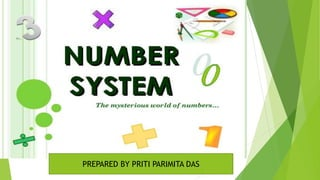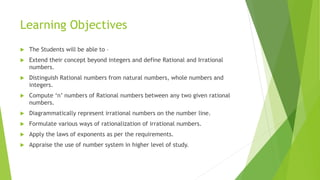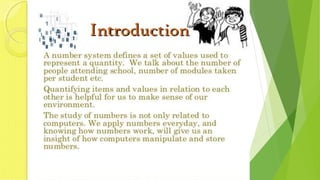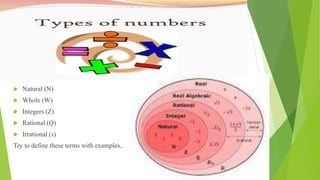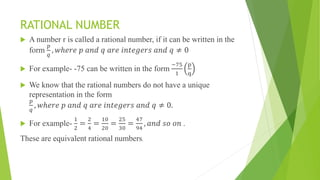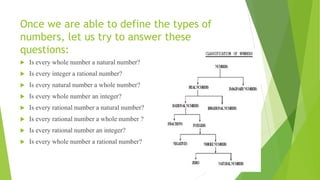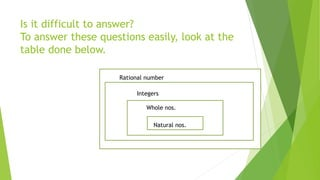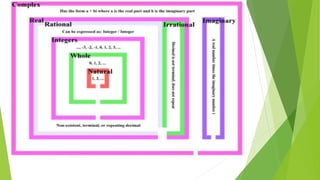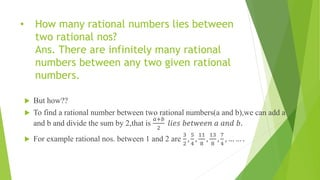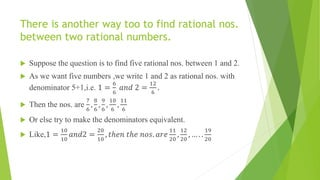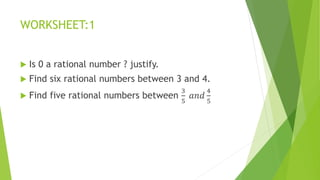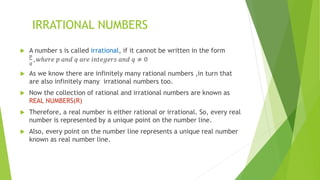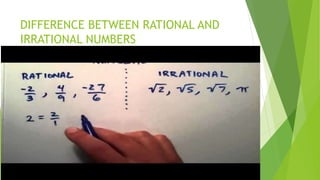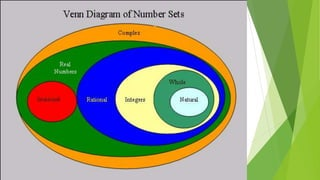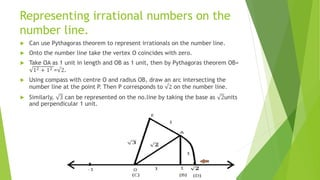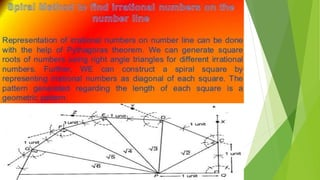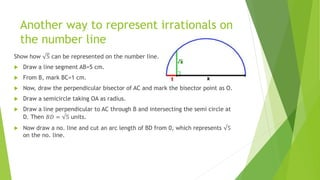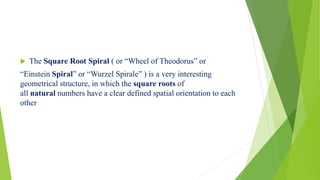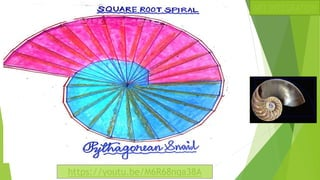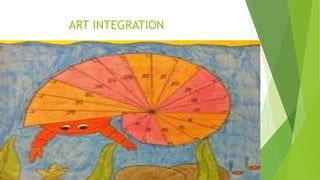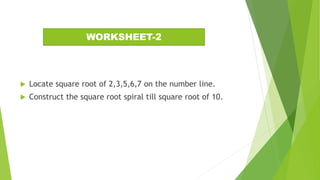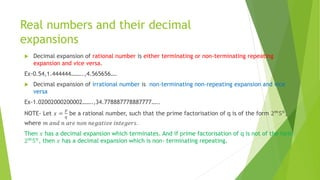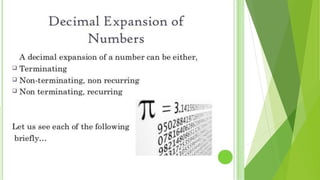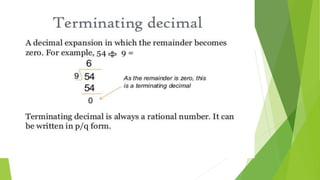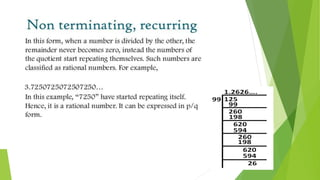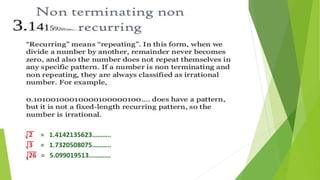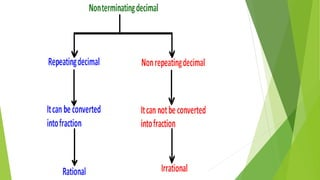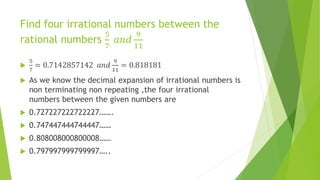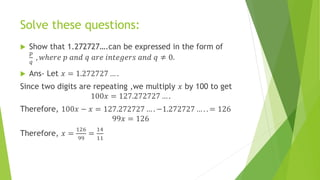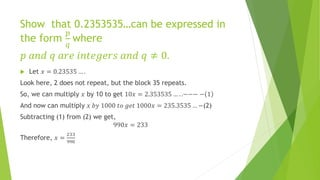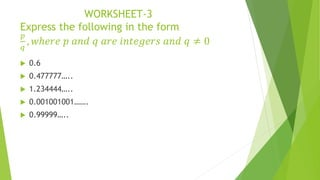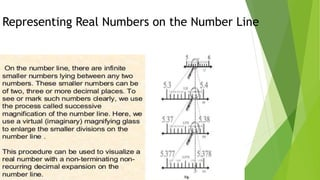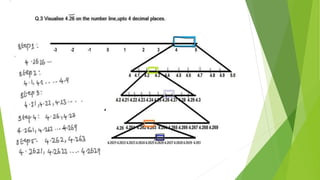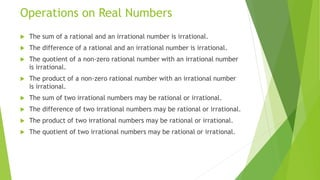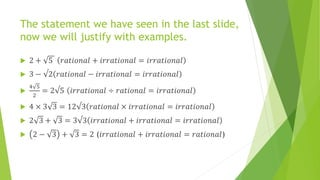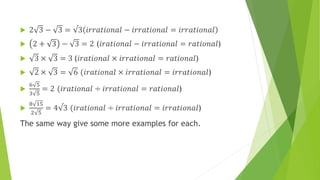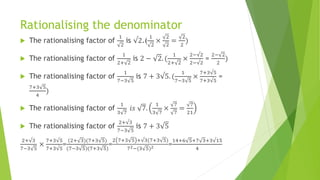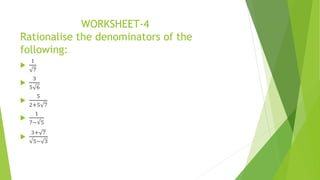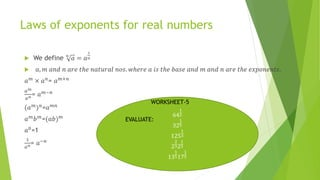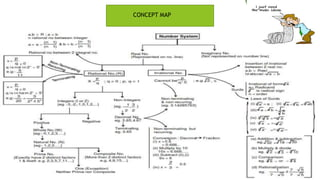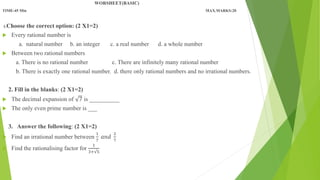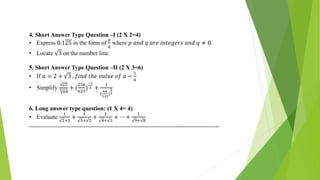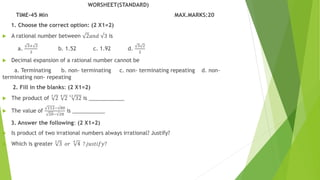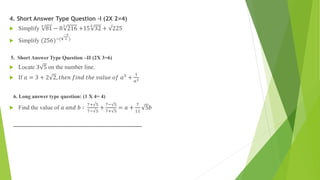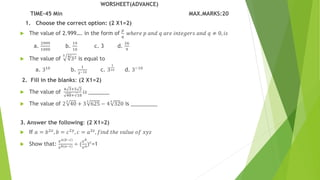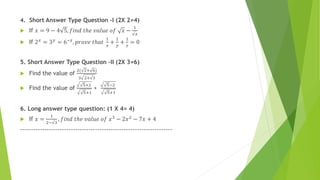1 de 48

### ix-number system-ppt(2).pptx

• 1. DAV PUBLIC SCHOOL,POKHARIPUT,BHUBANESWAR DERARTMENT OF MATHEMATICS CLASS-IX MAT HS DAV INSTITUTIONS ,ODISHA ZONE-1
• 2. PREPARED BY PRITI PARIMITA DAS
• 3. Learning Objectives  The Students will be able to –  Extend their concept beyond integers and define Rational and Irrational numbers.  Distinguish Rational numbers from natural numbers, whole numbers and integers.  Compute ‘n’ numbers of Rational numbers between any two given rational numbers.  Diagrammatically represent irrational numbers on the number line.  Formulate various ways of rationalization of irrational numbers.  Apply the laws of exponents as per the requirements.  Appraise the use of number system in higher level of study.
• 5.  Natural (N)  Whole (W)  Integers (Z)  Rational (Q)  Irrational (s) Try to define these terms with examples.
• 6. RATIONAL NUMBER  A number r is called a rational number, if it can be written in the form 𝑝 𝑞 , 𝑤ℎ𝑒𝑟𝑒 𝑝 𝑎𝑛𝑑 𝑞 𝑎𝑟𝑒 𝑖𝑛𝑡𝑒𝑔𝑒𝑟𝑠 𝑎𝑛𝑑 𝑞 ≠ 0  For example- -75 can be written in the form −75 1 p q  We know that the rational numbers do not have a unique representation in the form 𝑝 𝑞 , 𝑤ℎ𝑒𝑟𝑒 𝑝 𝑎𝑛𝑑 𝑞 𝑎𝑟𝑒 𝑖𝑛𝑡𝑒𝑔𝑒𝑟𝑠 𝑎𝑛𝑑 𝑞 ≠ 0.  For example- 1 2 = 2 4 = 10 20 = 25 30 = 47 94 , 𝑎𝑛𝑑 𝑠𝑜 𝑜𝑛 . These are equivalent rational numbers.
• 7. Once we are able to define the types of numbers, let us try to answer these questions:  Is every whole number a natural number?  Is every integer a rational number?  Is every natural number a whole number?  Is every whole number an integer?  Is every rational number a natural number?  Is every rational number a whole number ?  Is every rational number an integer?  Is every whole number a rational number?
• 8. Is it difficult to answer? To answer these questions easily, look at the table done below. n Rational number Integers Whole nos. n Natural nos.
• 10. • How many rational numbers lies between two rational nos? Ans. There are infinitely many rational numbers between any two given rational numbers.  But how??  To find a rational number between two rational numbers(a and b),we can add a and b and divide the sum by 2,that is 𝑎+𝑏 2 𝑙𝑖𝑒𝑠 𝑏𝑒𝑡𝑤𝑒𝑒𝑛 𝑎 𝑎𝑛𝑑 𝑏.  For example rational nos. between 1 and 2 are 3 2 , 5 4 , 11 8 , 13 8 , 7 4 , … … .
• 11. There is another way too to find rational nos. between two rational numbers.  Suppose the question is to find five rational nos. between 1 and 2.  As we want five numbers ,we write 1 and 2 as rational nos. with denominator 5+1,i.e. 1 = 6 6 𝑎𝑛𝑑 2 = 12 6 .  Then the nos. are 7 6 , 8 6 , 9 6 , 10 6 , 11 6  Or else try to make the denominators equivalent.  Like,1 = 10 10 𝑎𝑛𝑑2 = 20 10 , 𝑡ℎ𝑒𝑛 𝑡ℎ𝑒 𝑛𝑜𝑠. 𝑎𝑟𝑒 11 20 , 12 20 , … . . 19 20
• 12. WORKSHEET:1  Is 0 a rational number ? justify.  Find six rational numbers between 3 and 4.  Find five rational numbers between 3 5 𝑎𝑛𝑑 4 5
• 13. IRRATIONAL NUMBERS  A number s is called irrational, if it cannot be written in the form 𝑝 𝑞 , 𝑤ℎ𝑒𝑟𝑒 𝑝 𝑎𝑛𝑑 𝑞 𝑎𝑟𝑒 𝑖𝑛𝑡𝑒𝑔𝑒𝑟𝑠 𝑎𝑛𝑑 𝑞 ≠ 0  As we know there are infinitely many rational numbers ,in turn that are also infinitely many irrational numbers too.  Now the collection of rational and irrational numbers are known as REAL NUMBERS(R)  Therefore, a real number is either rational or irrational. So, every real number is represented by a unique point on the number line.  Also, every point on the number line represents a unique real number known as real number line.
• 14. DIFFERENCE BETWEEN RATIONAL AND IRRATIONAL NUMBERS
• 16. Representing irrational numbers on the number line.  Can use Pythagoras theorem to represent irrationals on the number line.  Onto the number line take the vertex O coincides with zero.  Take OA as 1 unit in length and OB as 1 unit, then by Pythagoras theorem OB= 12 + 12 =√2.  Using compass with centre O and radius OB, draw an arc intersecting the number line at the point P. Then P corresponds to √2 on the number line.  Similarly, 3 can be represented on the no.line by taking the base as 2units and perpendicular 1 unit.
• 18. Another way to represent irrationals on the number line Show how 5 can be represented on the number line.  Draw a line segment AB=5 cm.  From B, mark BC=1 cm.  Now, draw the perpendicular bisector of AC and mark the bisector point as O.  Draw a semicircle taking OA as radius.  Draw a line perpendicular to AC through B and intersecting the semi circle at D. Then 𝐵𝐷 = √5 units.  Now draw a no. line and cut an arc length of BD from 0, which represents √5 on the no. line.
• 19.  The Square Root Spiral ( or “Wheel of Theodorus” or “Einstein Spiral” or “Wurzel Spirale” ) is a very interesting geometrical structure, in which the square roots of all natural numbers have a clear defined spatial orientation to each other
• 22.  Locate square root of 2,3,5,6,7 on the number line.  Construct the square root spiral till square root of 10. WORKSHEET-2
• 23. Real numbers and their decimal expansions  Decimal expansion of rational number is either terminating or non-terminating repeating expansion and vice versa. Ex-0.54,1.444444……..,4.565656….  Decimal expansion of irrational number is non-terminating non-repeating expansion and vice versa Ex-1.02002000200002…….,34.778887778887777….. NOTE- Let 𝑥 = 𝑝 𝑞 be a rational number, such that the prime factorisation of q is of the form 2𝑚5𝑛, where 𝑚 𝑎𝑛𝑑 𝑛 𝑎𝑟𝑒 𝑛𝑜𝑛 𝑛𝑒𝑔𝑎𝑡𝑖𝑣𝑒 𝑖𝑛𝑡𝑒𝑔𝑒𝑟𝑠. Then 𝑥 has a decimal expansion which terminates. And if prime factorisation of q is not of the form 2𝑚 5𝑛 , then 𝑥 has a decimal expansion which is non- terminating repeating.
• 29. Find four irrational numbers between the rational numbers 5 7 𝑎𝑛𝑑 9 11  5 7 = 0.7142857142 𝑎𝑛𝑑 9 11 = 0.818181  As we know the decimal expansion of irrational numbers is non terminating non repeating ,the four irrational numbers between the given numbers are  0.727227222722227…….  0.747447444744447……  0.808008000800008……  0.797997999799997…..
• 30. Solve these questions:  Show that 1.272727….can be expressed in the form of 𝑝 𝑞 , 𝑤ℎ𝑒𝑟𝑒 𝑝 𝑎𝑛𝑑 𝑞 𝑎𝑟𝑒 𝑖𝑛𝑡𝑒𝑔𝑒𝑟𝑠 𝑎𝑛𝑑 𝑞 ≠ 0.  Ans- Let 𝑥 = 1.272727 … . Since two digits are repeating ,we multiply 𝑥 by 100 to get 100𝑥 = 127.272727 … . Therefore, 100𝑥 − 𝑥 = 127.272727 … . −1.272727 … . . = 126 99𝑥 = 126 Therefore, 𝑥 = 126 99 = 14 11
• 31. Show that 0.2353535…can be expressed in the form 𝑝 𝑞 where 𝑝 𝑎𝑛𝑑 𝑞 𝑎𝑟𝑒 𝑖𝑛𝑡𝑒𝑔𝑒𝑟𝑠 𝑎𝑛𝑑 𝑞 ≠ 0.  Let 𝑥 = 0.23535 … . Look here, 2 does not repeat, but the block 35 repeats. So, we can multiply 𝑥 by 10 to get 10𝑥 = 2.353535 … . .−−− − 1 And now can multiply 𝑥 𝑏𝑦 1000 𝑡𝑜 𝑔𝑒𝑡 1000𝑥 = 235.3535 … −(2) Subtracting (1) from (2) we get, 990𝑥 = 233 Therefore, 𝑥 = 233 990
• 32. WORKSHEET-3 Express the following in the form 𝑝 𝑞 , 𝑤ℎ𝑒𝑟𝑒 𝑝 𝑎𝑛𝑑 𝑞 𝑎𝑟𝑒 𝑖𝑛𝑡𝑒𝑔𝑒𝑟𝑠 𝑎𝑛𝑑 𝑞 ≠ 0  0.6  0.477777…..  1.234444…..  0.001001001…….  0.99999…..
• 33. Representing Real Numbers on the Number Line
• 35. Operations on Real Numbers  The sum of a rational and an irrational number is irrational.  The difference of a rational and an irrational number is irrational.  The quotient of a non-zero rational number with an irrational number is irrational.  The product of a non-zero rational number with an irrational number is irrational.  The sum of two irrational numbers may be rational or irrational.  The difference of two irrational numbers may be rational or irrational.  The product of two irrational numbers may be rational or irrational.  The quotient of two irrational numbers may be rational or irrational.
• 36. The statement we have seen in the last slide, now we will justify with examples.  2 + 5 𝑟𝑎𝑡𝑖𝑜𝑛𝑎𝑙 + 𝑖𝑟𝑟𝑎𝑡𝑖𝑜𝑛𝑎𝑙 = 𝑖𝑟𝑟𝑎𝑡𝑖𝑜𝑛𝑎𝑙  3 − √2 𝑟𝑎𝑡𝑖𝑜𝑛𝑎𝑙 − 𝑖𝑟𝑟𝑎𝑡𝑖𝑜𝑛𝑎𝑙 = 𝑖𝑟𝑟𝑎𝑡𝑖𝑜𝑛𝑎𝑙  4 5 2 = 2√5 𝑖𝑟𝑟𝑎𝑡𝑖𝑜𝑛𝑎𝑙 ÷ 𝑟𝑎𝑡𝑖𝑜𝑛𝑎𝑙 = 𝑖𝑟𝑟𝑎𝑡𝑖𝑜𝑛𝑎𝑙  4 × 3 3 = 12√3 𝑟𝑎𝑡𝑖𝑜𝑛𝑎𝑙 × 𝑖𝑟𝑟𝑎𝑡𝑖𝑜𝑛𝑎𝑙 = 𝑖𝑟𝑟𝑎𝑡𝑖𝑜𝑛𝑎𝑙  2 3 + 3 = 3√3 𝑖𝑟𝑟𝑎𝑡𝑖𝑜𝑛𝑎𝑙 + 𝑖𝑟𝑟𝑎𝑡𝑖𝑜𝑛𝑎𝑙 = 𝑖𝑟𝑟𝑎𝑡𝑖𝑜𝑛𝑎𝑙  2 − 3 + 3 = 2 (𝑖𝑟𝑟𝑎𝑡𝑖𝑜𝑛𝑎𝑙 + 𝑖𝑟𝑟𝑎𝑡𝑖𝑜𝑛𝑎𝑙 = 𝑟𝑎𝑡𝑖𝑜𝑛𝑎𝑙)
• 37.  2 3 − 3 = √3 𝑖𝑟𝑟𝑎𝑡𝑖𝑜𝑛𝑎𝑙 − 𝑖𝑟𝑟𝑎𝑡𝑖𝑜𝑛𝑎𝑙 = 𝑖𝑟𝑟𝑎𝑡𝑖𝑜𝑛𝑎𝑙  2 + 3 − 3 = 2 (𝑖𝑟𝑎𝑡𝑖𝑜𝑛𝑎𝑙 − 𝑖𝑟𝑟𝑎𝑡𝑖𝑜𝑛𝑎𝑙 = 𝑟𝑎𝑡𝑖𝑜𝑛𝑎𝑙)  3 × 3 = 3 (𝑖𝑟𝑎𝑡𝑖𝑜𝑛𝑎𝑙 × 𝑖𝑟𝑟𝑎𝑡𝑖𝑜𝑛𝑎𝑙 = 𝑟𝑎𝑡𝑖𝑜𝑛𝑎𝑙)  2 × 3 = 6 (𝑖𝑟𝑎𝑡𝑖𝑜𝑛𝑎𝑙 × 𝑖𝑟𝑟𝑎𝑡𝑖𝑜𝑛𝑎𝑙 = 𝑖𝑟𝑟𝑎𝑡𝑖𝑜𝑛𝑎𝑙)  6 5 3 5 = 2 (𝑖𝑟𝑎𝑡𝑖𝑜𝑛𝑎𝑙 ÷ 𝑖𝑟𝑟𝑎𝑡𝑖𝑜𝑛𝑎𝑙 = 𝑟𝑎𝑡𝑖𝑜𝑛𝑎𝑙)  8 15 2 5 = 4√3 (𝑖𝑟𝑎𝑡𝑖𝑜𝑛𝑎𝑙 ÷ 𝑖𝑟𝑟𝑎𝑡𝑖𝑜𝑛𝑎𝑙 = 𝑖𝑟𝑟𝑎𝑡𝑖𝑜𝑛𝑎𝑙) The same way give some more examples for each.
• 38. Rationalising the denominator  The rationalising factor of 1 2 is √2.( 1 2 × 2 2 = 2 2 )  The rationalising factor of 1 2+ 2 is 2 − 2. ( 1 2+ 2 × 2−√2 2−√2 = 2− 2 2 )  The rationalising factor of 1 7−3 5 is 7 + 3√5. ( 1 7−3 5 × 7+3√5 7+3√5 = 7+3 5 4 )  The rationalising factor of 1 3 7 𝑖𝑠 7. 1 3 7 × 7 7 = 7 21  The rationalising factor of 2+√3 7−3 5 is 7 + 3 5 2+√3 7−3 5 × 7+3√5 7+3√5 = (2+ 3)(7+3 5) (7−3 5)(7+3 5) = 2 7+3 5 +√3(7+3 5) 72−(3 5)2 = 14+6 5+7 3+3√15 4
• 39. WORKSHEET-4 Rationalise the denominators of the following:  1 7  3 5 6  5 2+5 7  1 7−√5  3+ 7 √5− 3
• 40. Laws of exponents for real numbers  We define 𝑛 𝑎 = 𝑎 1 𝑛  𝑎, 𝑚 𝑎𝑛𝑑 𝑛 𝑎𝑟𝑒 𝑡ℎ𝑒 𝑛𝑎𝑡𝑢𝑟𝑎𝑙 𝑛𝑜𝑠. 𝑤ℎ𝑒𝑟𝑒 𝑎 𝑖𝑠 𝑡ℎ𝑒 𝑏𝑎𝑠𝑒 𝑎𝑛𝑑 𝑚 𝑎𝑛𝑑 𝑛 𝑎𝑟𝑒 𝑡ℎ𝑒 𝑒𝑥𝑝𝑜𝑛𝑒𝑛𝑡𝑠. 𝑎𝑚 × 𝑎𝑛 = 𝑎𝑚+𝑛 𝑎𝑚 𝑎𝑛 = 𝑎𝑚−𝑛 (𝑎𝑚)𝑛=𝑎𝑚𝑛 𝑎𝑚𝑏𝑚=(𝑎𝑏)𝑚 𝑎0 =1 1 𝑎𝑛= 𝑎−𝑛 64 1 2 32 1 5 125 1 3 2 2 32 1 5 13 1 517 1 5 EVALUATE: WORKSHEET-5
• 42. WORSHEET(BASIC) TIME-45 Min MAX.MARKS:20 1.Choose the correct option: (2 X1=2)  Every rational number is a. natural number b. an integer c. a real number d. a whole number  Between two rational numbers a. There is no rational number c. There are infinitely many rational number b. There is exactly one rational number. d. there only rational numbers and no irrational numbers. 2. Fill in the blanks: (2 X1=2)  The decimal expansion of 7 is __________  The only even prime number is ___ 3. Answer the following: (2 X1=2)  Find an irrational number between 1 7 𝑎𝑛𝑑 2 7  Find the rationalising factor for 1 3+√5
• 43. 4. Short Answer Type Question –I (2 X 2=4) • Express 0.125 in the form of 𝑝 𝑞 where 𝑝 𝑎𝑛𝑑 𝑞 𝑎𝑟𝑒 𝑖𝑛𝑡𝑒𝑔𝑒𝑟𝑠 𝑎𝑛𝑑 𝑞 ≠ 0. • Locate 3 on the number line. 5. Short Answer Type Question –II (2 X 3=6) • If 𝑎 = 2 + 3 , 𝑓𝑖𝑛𝑑 𝑡ℎ𝑒 𝑣𝑎𝑙𝑢𝑒 𝑜𝑓 𝑎 − 1 𝑎 • Simplify 25 3 64 + ( 256 625 ) −1 4 + 1 ( 64 125 ) 2 3 6. Long answer type question: (1 X 4= 4) • Evaluate 1 2+1 + 1 3+√2 + 1 4+√3 + ⋯ + 1 9+√8 -----------------------------------------------------------------------------------------------
• 44. WORSHEET(STANDARD) TIME-45 Min MAX.MARKS:20 1. Choose the correct option: (2 X1=2)  A rational number between 2𝑎𝑛𝑑 √3 is a. 3+ 2 2 b. 1.52 c. 1.92 d. 3 2 2  Decimal expansion of a rational number cannot be a. Terminating b. non- terminating c. non- terminating repeating d. non- terminating non- repeating 2. Fill in the blanks: (2 X1=2)  The product of 3 2 4 2 12 32 is ____________  The value of 112−√80 20−√28 is ___________ 3. Answer the following: (2 X1=2)  Is product of two irrational numbers always irrational? Justify?  Which is greater 3 3 𝑜𝑟 4 4 ? 𝑗𝑢𝑠𝑡𝑖𝑓𝑦?
• 45. 4. Short Answer Type Question –I (2X 2=4)  Simplify 4 81 − 8 3 216 +15 5 32 + √225  Simplify (256)−(4 −3 2 ) 5. Short Answer Type Question –II (2X 3=6)  Locate 3 5 on the number line.  If 𝑎 = 3 + 2 2, 𝑡ℎ𝑒𝑛 𝑓𝑖𝑛𝑑 𝑡ℎ𝑒 𝑣𝑎𝑙𝑢𝑒 𝑜𝑓 𝑎3 + 1 𝑎3 6. Long answer type question: (1 X 4= 4)  Find the value of 𝑎 𝑎𝑛𝑑 𝑏 ∶ 7+√5 7−√5 + 7−√5 7+√5 = 𝑎 + 7 11 5𝑏 ------------------------------------------------------------------------
• 46. WORSHEET(ADVANCE) TIME-45 Min MAX.MARKS:20 1. Choose the correct option: (2 X1=2)  The value of 2.999…. in the form of 𝑝 𝑞 𝑤ℎ𝑒𝑟𝑒 𝑝 𝑎𝑛𝑑 𝑞 𝑎𝑟𝑒 𝑖𝑛𝑡𝑒𝑔𝑒𝑟𝑠 𝑎𝑛𝑑 𝑞 ≠ 0, 𝑖𝑠 a. 2999 1000 b. 19 10 c. 3 d. 26 9  The value of 5 ∜32 is equal to a. 310 b. 1 3−10 c. 3 1 10 d. 3−10 2. Fill in the blanks: (2 X1=2)  The value of 4 3+5 2 48+√18 𝑖𝑠 __________  The value of 2 3 40 + 3 3 625 − 4 3 320 is _________ 3. Answer the following: (2 X1=2)  If 𝑎 = 𝑏2𝑥 , 𝑏 = 𝑐2𝑦 , 𝑐 = 𝑎2𝑧 , 𝑓𝑖𝑛𝑑 𝑡ℎ𝑒 𝑣𝑎𝑙𝑢𝑒 𝑜𝑓 𝑥𝑦𝑧  Show that: 𝑥𝑎(𝑏−𝑐) 𝑥𝑏(𝑎−𝑐) ÷ ( 𝑥𝑏 𝑥𝑎)𝑐 =1
• 47. 4. Short Answer Type Question –I (2X 2=4)  If 𝑥 = 9 − 4 5, 𝑓𝑖𝑛𝑑 𝑡ℎ𝑒 𝑣𝑎𝑙𝑢𝑒 𝑜𝑓 𝑥 − 1 √𝑥  If 2𝑥 = 3𝑦 = 6−𝑧 , 𝑝𝑟𝑜𝑣𝑒 𝑡ℎ𝑎𝑡 1 𝑥 + 1 𝑦 + 1 𝑧 = 0 5. Short Answer Type Question –II (2X 3=6)  Find the value of 2( 2+ 6) 3 2+√3  Find the value of 5+2 5+1 + 5−2 5+1 6. Long answer type question: (1 X 4= 4)  If 𝑥 = 1 2−√3 , 𝑓𝑖𝑛𝑑 𝑡ℎ𝑒 𝑣𝑎𝑙𝑢𝑒 𝑜𝑓 𝑥3 − 2𝑥2 − 7𝑥 + 4 ---------------------------------------------------------------------